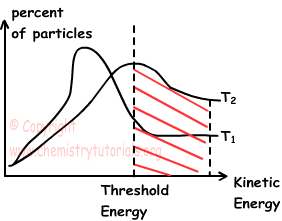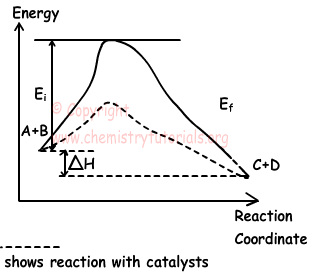EN | ES | DE | NL | RU

## Factors Affecting Reaction Rate

Factors Affecting Reaction Rate

In last section, we give factors affecting reaction rate. Now, we learn them in detail one by one.

1. Types of matter in reaction:

• In general reactions having reactants and products in gas phases have higher reaction rate than reactions consisting of matters in liquid phase.
• Breaking too many bonds and forming new ones makes reaction slow down.
• Reactions consisting of ions having opposite signs have high reaction rate.

2. Concentration of matters in reaction:

Collision theory is the most successful one that explains chemical reaction. Particles must collide to react each other. On the contrary, all collisions do not result with reaction.

• Matters should have required energy to react.
• Particles should collide in appropriate geometry to react.

Relation between concentrations of matters and reaction rate can be explained like;

aA  + bB cC + dD

Reaction rate of this reaction is found by;

Reaction Rate = k. [A]a.[B]b

Where; k is the rate constant or rate coefficient that depends on temperature and a and b are exponents.

Order of Reaction;

Order of reaction is the sum of exponents. For example;

Reaction Rate = k. [A] order of this reaction is 1.

Reaction Rate = k. [A].[B] order of this reaction is 1+1=2.

Reaction Rate = k. [A]2.[B].[C]3 order of this reaction is 2+1+3=6.

Reaction Mechanism

Rate of reaction is determined by the slower step of reaction.What we mean by slower step is explained with following example;

NO2(g) + CO(g) → CO2(g) + NO(g)

Reaction rate of this reaction should be;

Reaction Rate= k. [NO2].[CO]

O the contrary, experiments done on this reaction shows that reaction rate is;

Reaction Rate= k. [NO2]2

This situation shows that reaction takes place step by step. We can write parts of this reaction as;

I. 2NO2(g) → NO3(g) + NO(g)    Slow

II. NO3(g) + CO(g) → CO2(g) + NO2(g)  Fast

As you can see from the steps, reaction rate is determined by slow reaction. Using I. reaction we write;

Reaction Rate= k. [NO2]2

To sum up if reaction occurs in one step or slow step of two or more than two step reactions is taken into consideration and reaction rate is written.

aA + bB → Products

Reaction Rate = k.[A]a.[B]b

3.Temperature in Reactions:

Increasing in the temperature about 10 0C, results increase in the reaction rate. When we increase temperature;

• number of collisions in unit time increases
• number of particles having energy above activation energy increases.

In graph given below, collisions that results in reaction are shown with striped part.On the contrary, if we give values in two different temperature, graph becomes;As you can see from the graph, as we increase temperature, number of particles passing threshold energy increases which also results in increase reaction rate.

4. Catalysts:

Catalysts are matters that increase or decrease reaction rate and they do not being changed in reaction. Graph given below shows effect of catalysts on reaction.ΔH = Ei-Ef

Catalysts are used to increase or decrease reaction rate.

5. Surface of Reactants:

If size of particles are small, then surface of them increases. Increasing surface of the reactants, increase reaction rate.

Example: Which ones of the following applications increases rate of reaction in gas phase?

II. Decreasing pressure

III. Increasing temperature

IV. Increasing surface area of reactants

Solution:

Adding catalysts decrease threshold energy and increase reaction rate. Increasing temperature and surface area of reactants also increase reaction rate. On the contrary decreasing pressure increases volume and number of collisions decrease. So, decreasing pressure decreases reaction rate.

The Original Author: